# Category

A concept formalizing a number of algebraic properties of collections of morphism between mathematical objects of the same type (sets, topological spaces, groups, etc.) under the condition that these collections contain the identity mappings and are closed with respect to successive composition (or product) of mappings. A category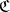consists of a class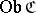, whose elements are called objects of the category, and a class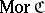, the elements of which are called morphisms of the category. These classes must satisfy the following conditions:

1) to each ordered pair of objects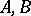is associated a set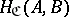(also denoted by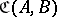,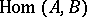, or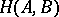) of members of. If, thenis called the source, or domain (of definition), of the morphism, andthe target, or range (of values), or codomain of; instead of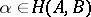one often writesor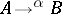;

2) each morphism of the categorybelongs to only one set;

3) in the class, the following partial composition (product) rule is given: The product of two morphismsand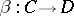is defined if and only if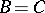, in which case it belongs to; the product ofandis denoted by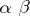, or, depending on one's choice of conventions, by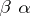;

4) for any morphisms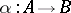,and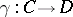, the associativity law holds: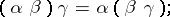5) each set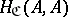contains a (distinguished) morphism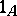such thatandfor any morphisms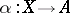and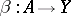; the morphismsare called units, identities or ones.

The notion of a class occurring in the definition of a category presupposes the use of axioms from set theory which distinguish between sets and classes. The most commonly used is the axiom scheme of Gödel–Bernays–von Neumann.

Sometimes, in the definition of a category, it is not required that the classesbe sets. Sometimes, instead of using classes, one assumes the existence of a universal set and requires that the classesandbelong to a fixed universal set.

Since there is a bijective correspondence between the identities of a categoryand the class, a categorycan be defined as a class of morphisms with a partial product satisfying additional conditions (see, for example, , ).

The notion of a category was introduced in 1945 . The origins of category theory and the initial stimulus for its development came from algebraic topology. Subsequent investigation revealed the unifying role of the notion of a category and the notion of a functor related to it for many branches of mathematics.

## Contents

### Examples of categories.

1) The category of sets. The class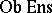consists of all sets; the classconsists of all functions between sets; composition of morphism is composition of functions (see Sets, category of).

2) The category of topological spaces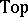(or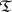). The class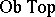consists of all topological spaces, the classof all continuous mappings, while composition is again composition of mappings.

3) The category of groups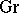(or). The class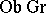consists of all groups, the class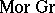of all group homomorphisms, and composition again is composition of homomorphisms (see Category of groups). One defines in a similar fashion the categories of vector spaces over some field, the category of rings, etc.

4) The category of binary relations between sets(or). The class of objects of this category is, but as morphisms fromtoone takes all binary relations, that is, all subsets of the Cartesian product; composition is composition of binary relations (cf. Binary relation).

5) A monoid (semi-group with identity) is a category with a single object; conversely, every category consisting of a single object is a monoid.

6) A pre-ordered set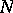can be regarded as a categoryfor which,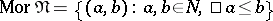, while the product is defined by the equality.

All categories listed above admit an "isomorphic imbedding" into the category of sets. A category with this property is called a concrete category. Not every category is concrete; for example, the category with as objects all topological spaces and whose morphisms are defined to be homotopy classes of continuous mappings .

The number of examples of categories can be considerably enlarged by means of various constructions; foremost, by means of functors of categories or categories of diagrams.

A mapping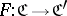between two categories,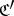is called a covariant functor (cf. also Functor) if for each object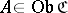,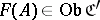, for each morphism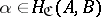, its image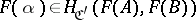, if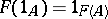, and if, finally,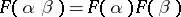whenever the productis defined. If the objects ofconstitute a set, then one can construct the category of diagramsor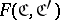, the objects of which are all covariant functors fromtoand with as morphisms all natural transformations of these functors.

To each categoryone can associate its dual category, or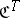, or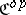, for whichand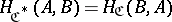for any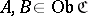. A covariant functor from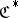tois called a contravariant functor fromto. Along with functors of one argument one can consider many-placed functors or functors of several arguments; cf. Functor.

For each statement in category theory there is a dual statement, obtained by a formal "reversal of the arrows" . In this connection the so-called duality principle holds: A statementis true in category theory if and only if the dual statement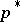is true.

Many concepts and results in mathematics turn out to be dual to others from a category-theoretic point of view: injectivity and projectivity, nilpotency and the notion of category of a topological space in the sense of Lyusternik–Shnirel'man, varieties and radicals in algebra, etc.

A category-theoretic analysis of the foundations of homology theory led to the introduction in the mid-fifties of so-called Abelian categories (cf. Abelian category). Within this framework it proved possible to realize the basic constructions of homological algebra . In the 1960s, interest in non-Abelian categories grew, as a result of problems in logic, general algebra, topology, and algebraic geometry. An intensive development of universal algebra and the axiomatic construction of homotopy theory marked the beginnings of various lines of investigation: the category-theoretic study of varieties of universal algebras, the theory of isomorphisms of direct decompositions, the theory of adjoint functors, and the theory of duality of functors. The latter development uncovered the existence of relations between these areas of study. As a result of the recent theory of relative categories, which makes wide use of the techniques of adjoint functors and closed categories, a duality has been established between homotopy theory and the theory of universal algebras; this is based on the interpretation of the categoric definitions of a monoid and a comonoid in suitable categories of functors (see, for example, ). Along with the development of the general theory of relative categories special classes of such categories were introduced:-categories or formal categories; categories with an involution or-categories, including, in particular, the category of binary relations; etc. A special case of-categories is the category of small categories, which can be placed at the basis of an axiomatic construction of mathematics.

The classes of categories listed above are characterized by the fact that their sets of morphismspossess an additional structure. Another method of introducing an additional structure in a category is to provide the category with a Grothendieck topology and to construct the category of sheaves over the topologized category (so-called topoi; cf. Topos).